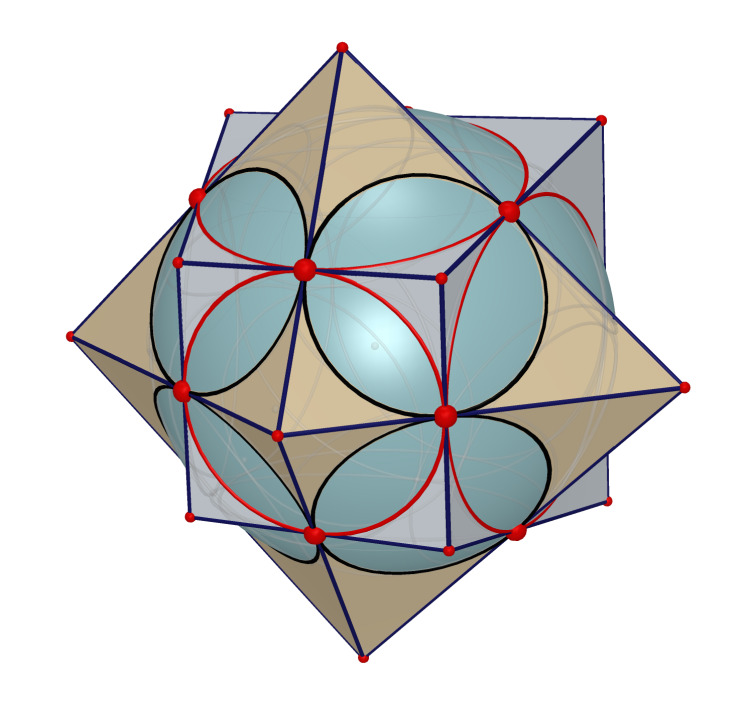Catalan Solid and Its Generalization

Jen-chung Chuan

Introduction

A Catalan solid is a dual polyhedron to an Archimedean solid. A list of 13 Catalan solids together with their Archimedean duals can be found in Wikipedia: https://en.wikipedia.org/wiki/Catalan_solid In this talk, we are to show the most efficient ways to construct all 13 dual pairs of polyhedrons, under the environment provided by the dynamic solid geometry software Cabri 3D. We then proceed to show with animations how each of such dual pairs can be distorted to form other generalized dual-pair polyhedrons families. Here we adopt the notion that two polyhedrons A and B are said to form a (generalized) dual pair if they have the same number of edges and each edge of A intersects a unique edge of B orthogonally in space.

Results

 Archimedean Solid Castalan Solid Duality Orthogonality Preserving Distortion­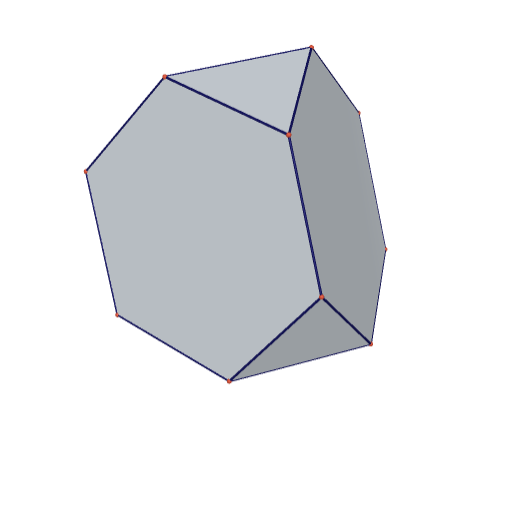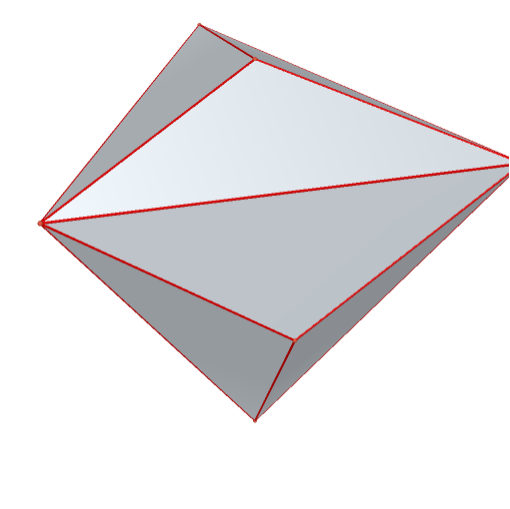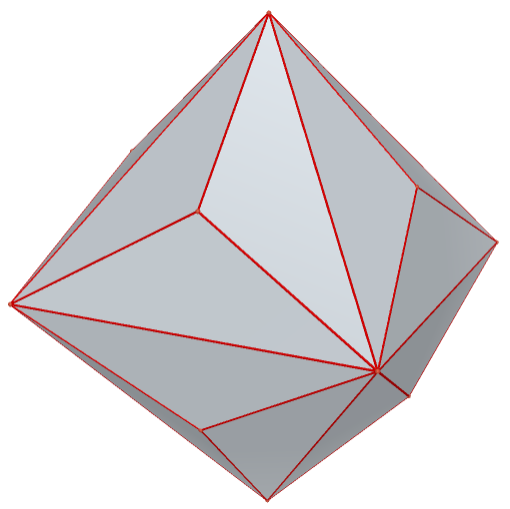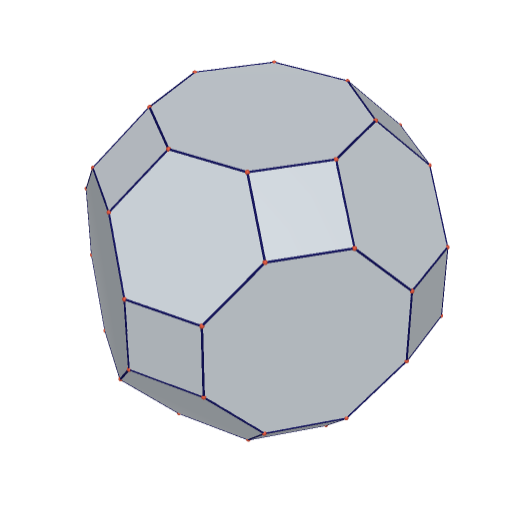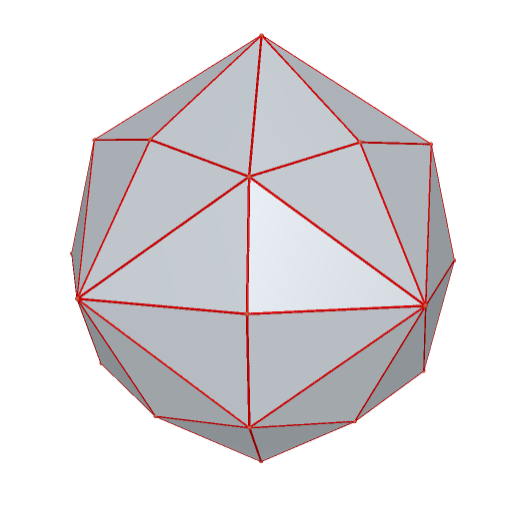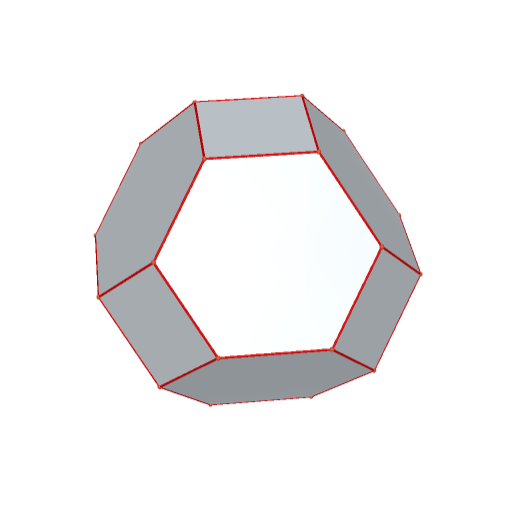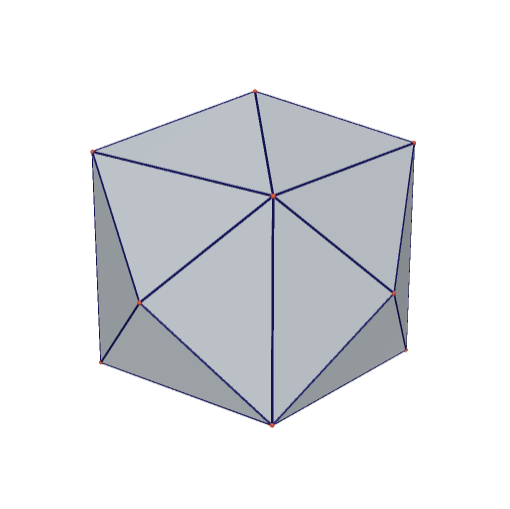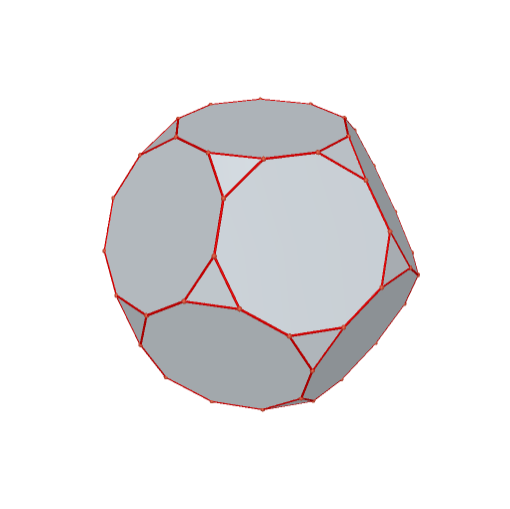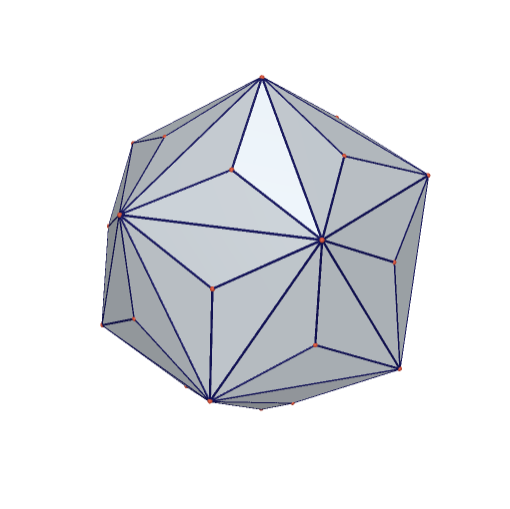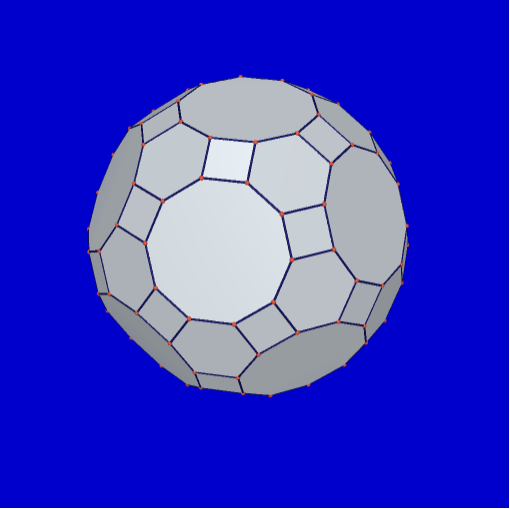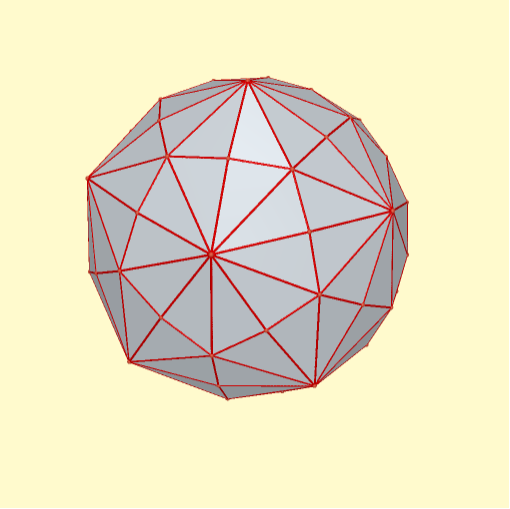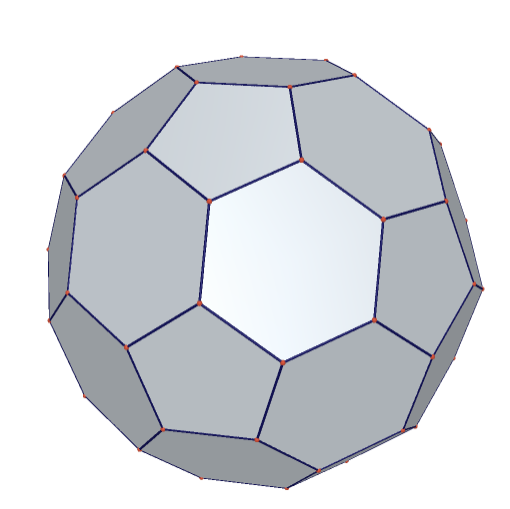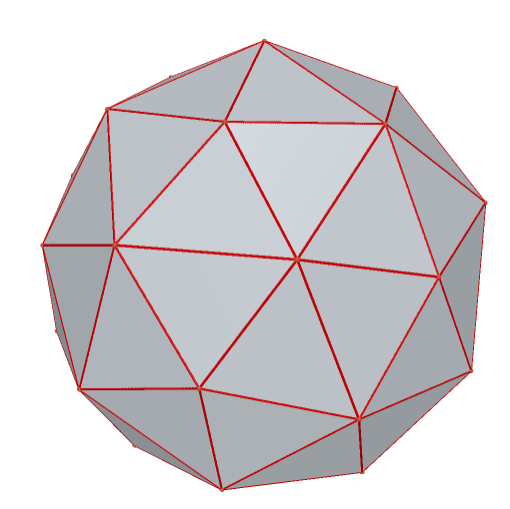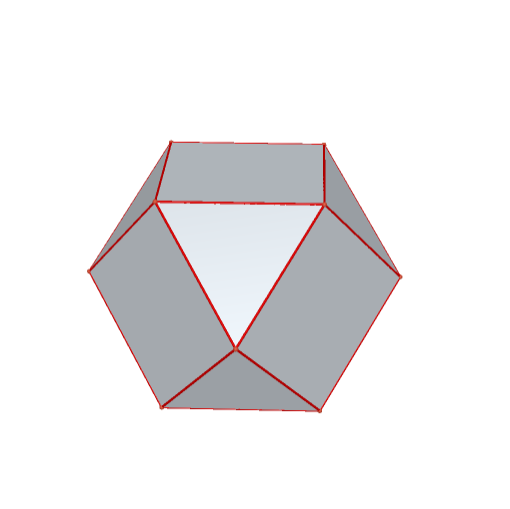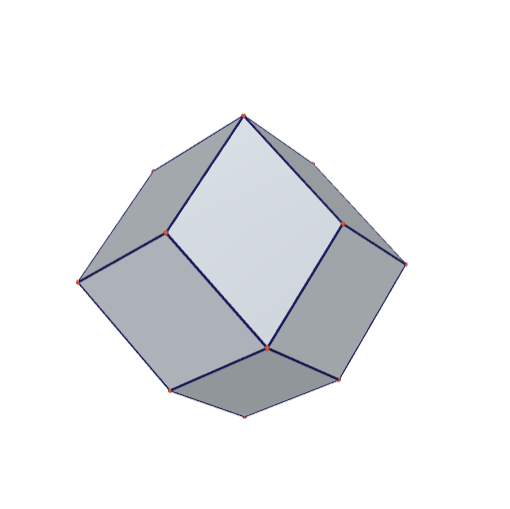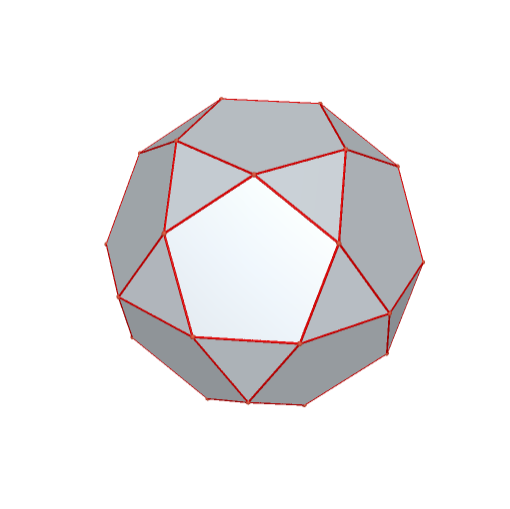icosidodecahedron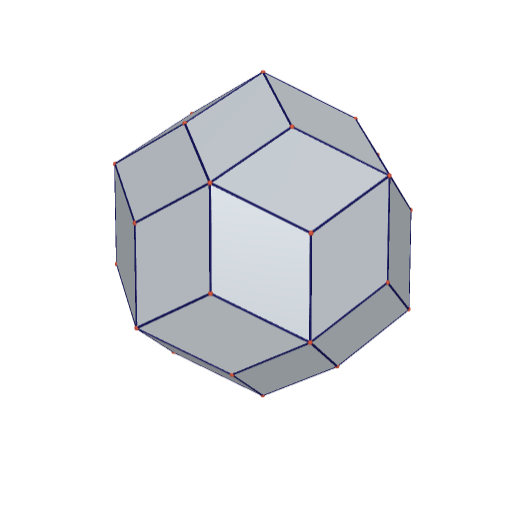snub tetrahedron pentagonal icositetrahedron

 Orthogonal Preserving Distortion of Regular Poyhedron and Its Dual Orthogonal Preserving Distortion of Tetrahedron and Its Dual Orthogonal Distortion of Cube-Octahedron Orthogonality Preserving Distortion of Dodecahedron-Icosahedron

 Orthogonal Preserving Distortion of Other Pairs Distortion Triangular Cupola Triangular Orthobicupola Triangular Orthobicupola-Trapezo-Rhombic Dodecahedron Distorted Symmetrically

Process of Distortion

Step 1. Construct the most symmetric pair of dual polyhedrons.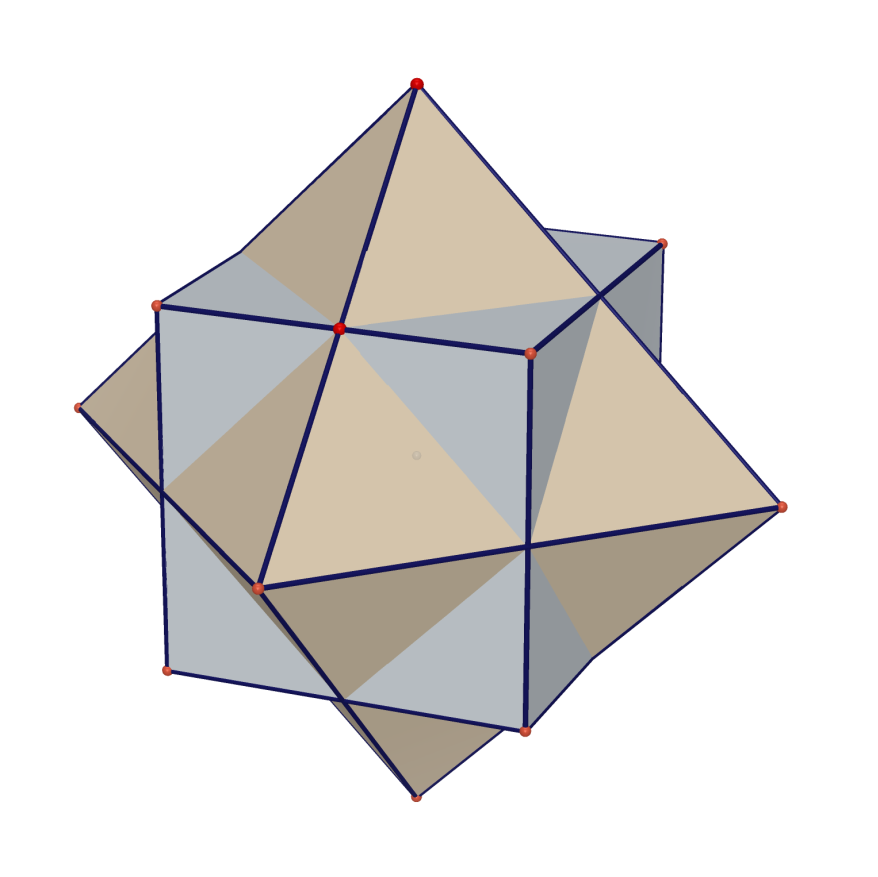Step 2. Since all edges are tangent to a same sphere S, their faces meet S in two orthogonal families of circles.1.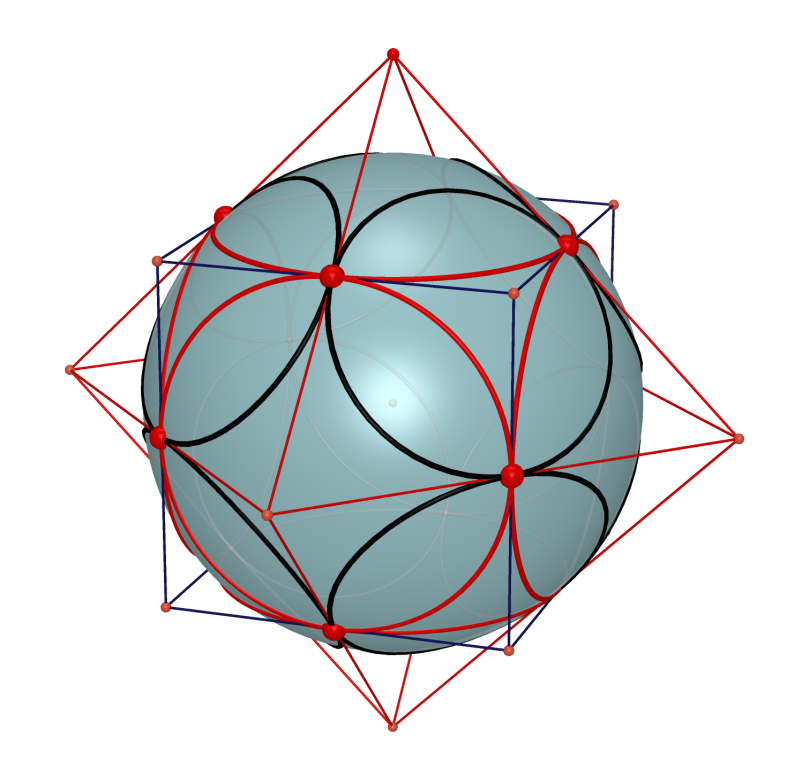Step 3. By taking inversion of circles with respect to S, two orthogonal families of circles are transformed to two new orthogonal families of circles.Step 4. Construct the two polyhedrons corresponding two the circles.